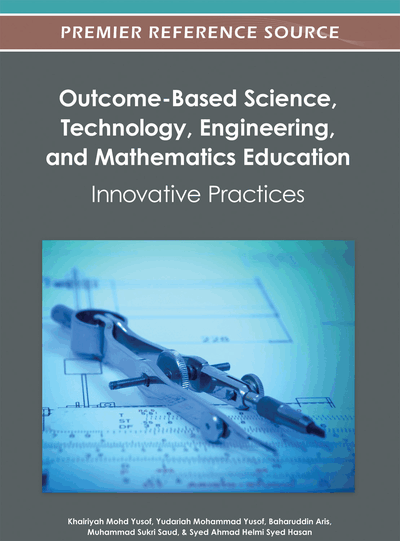# Designing a Blended Learning Model to Support Mathematical Thinking in Multivariable Calculus

Hamidreza Kashefi (Universiti Teknologi Malaysia, Malaysia), Zaleha Ismail (Universiti Teknologi Malaysia, Malaysia) and Yudariah Mohammad Yusof (Universiti Teknologi Malaysia, Malaysia)
DOI: 10.4018/978-1-4666-1809-1.ch011

## Abstract

Multivariable Calculus is one of the most difficult courses for undergraduate students. Researchers at Universiti Teknologi Malaysia (UTM) endeavor to support students in the learning of Multivariable Calculus by promoting mathematical thinking in a face-to-face classroom. This study attempts to identify not only learning difficulties in Multivariable Calculus through mathematical thinking approach but also the ways and means of improving these difficulties based on students and lecturers recommendations. The main purpose of this study is to establish a model of teaching and learning based on these findings for designing a blended learning instruction of Multivariable Calculus course through mathematical thinking approach. It first attempts to identify the theoretical reasons for selecting blended learning to promote mathematical thinking in Multivariable Calculus. Then, it exemplifies a teaching and learning model to support students’ mathematical thinking in Multivariable Calculus through blended learning instruction.
Chapter Preview
Top

## Introduction

Mathematics is a prime constituent and infrastructure of the education of undergraduate students. The application of calculus as the main analytical component of the syllabus in many fields shows calculus still plays a central role in undergraduate students’ education. Nowadays, calculus is one of the most important courses for undergraduate students and as such is offered as a prerequisite course to other advanced mathematics courses. However, calculus is one of the most difficult courses for most students to study in their field of study (Schwarzenberger, 1980; Tall, 1993a).

The learning difficulties of calculus for undergraduate students have been well documented. Some of these students’ difficulties in the learning of Basic Calculus are: the difficulty of learning some specific mathematical topics, the difficulty in coordinating procedures and manipulating concepts, the particular events in past experiences of students, poor problem solving skills, the inability to select and use appropriate mathematical representations, translating real-world problems into calculus formulations, absorbing complex new ideas in a limited time, and students’ beliefs and learning styles (Tall & Schwarzenberger, 1978; Orton, 1983a, b; Tall, 1993a; Yusof & Tall, 1999; Hirst, 2002; Rahman, 2009). There is not much literature dealing with the students’ difficulties in the learning of Multivariable Calculus. However, some students’ difficulties in Multivariable Calculus are common with students’ difficulties in Basic Calculus. Students’ difficulties in the specific topics, answering non-routine problems, poor mathematical knowledge background, poor ability in basic skills and algebraic manipulation, and students’ beliefs and learning styles are important challenges in the learning of Multivariable Calculus (Rahman, 2009; Kashefi, Zaleha & Yusof, 2010a, b).

The literature reviewed on students’ difficulties and the various efforts to improve the situations also showed a general trend, moving away from remedial classes towards teaching to increase understanding (Rahman, 2009). Improving students’ learning through the enhancement of their problem solving and mathematical thinking skills as well as through using technological tools to support conceptual understanding and problem solving methods are now thought to be more appropriate to enable them to cope with the mathematics needed for their fields of study. Researchers, by promoting mathematical thinking, endeavor to support students for understanding of mathematical concepts and solving real problems in face-to-face classrooms. Researchers like Tall (1986, 1989, 1990, 1993b, 1998, 2003) with computer, and researchers like Rahman and her colleagues (Rahman, 2009; Rahman, Yusof & Mason, 2007; Rahman, Yusof & Sabariah, 2007) without computer, try to support students to overcome their difficulties in the learning of Multivariable Calculus.

In this study, the ways of supporting students in the learning of calculus specifically in Multivariable Calculus are discussed. This study first attempt to uncover students’ difficulties in Multivariable Calculus through mathematical thinking method and look at ways of improving these difficulties based on the students’ and lecturers’ perspectives. Based on these findings, this study proposes blended learning as a teaching and learning environment to support students’ mathematical thinking and helps students to overcome their difficulties in Multivariable Calculus. The main purpose of this study is to establish a blended learning model that conceptualizes a framework for supporting mathematical knowledge construction and invoking students’ mathematical thinking powers and generic skills.

## Complete Chapter List

Search this Book:
Reset